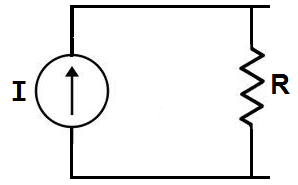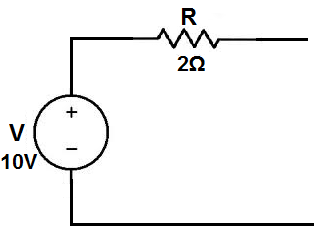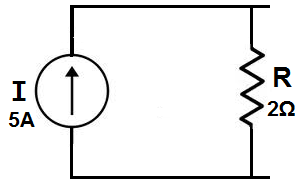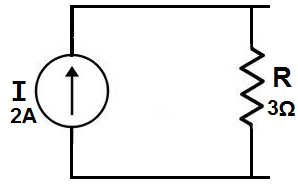﻿ Source Transformation of Circuits- Explained ﻿# Source Transformation of Circuits- ExplainedThe source transformation of a circuit is the transformation of a power source from a voltage source to a current source, or a current source to a voltage source.

In other words, we transform the power source from either voltage to current, or current to voltage.

### Voltage Source Transformation

We will first go over voltage source transformation, the transformation of a circuit with a voltage source to the equivalent circuit with a current source.

In order to get a visual example of this, let's take the circuit below which has a voltage source as its power source:Using source transformation, we can change or transform this above circuit with a voltage power source and a resistor, R, in series, into the equivalent circuit with a current source with a resistor, R, in parallel, as shown below:We transform a voltage source into a current source by using ohm's law. A voltage source can be changed into a current source by using ohm's formula, I=V/R.

### Example

Let's do an actual example to demonstrate the mathematics of ohm's law, using the circuit shown below:Here, we have a circuit with a voltage source of 10V with a resistor in series of 2Ω.

To calculate what the equivalent current source would be, we calculate it using the formula: I=V/R, which is I= 10V/2Ω= 5A. So the equivalent circuit would be:The new power source is now a 5A current source. The resistor value, however, as with all source transformations stays the same. The only thing that changes is it is now in parallel for a current source transformation.

Try out our calculator below. With this calculator, you can try out as many examples as you want. The calculator does source transformations and presents the new circuits with the new values.

### Current Source Transformation

We will now go over current source transformation, the transformation of a circuit with a current source to the equivalent circuit with a voltage source.

In order to get a visual example of this, let's take the circuit below which has a current source as its power source:Using source transformation, we can change or transform this above circuit with a current power source and a resistor, R, in parallel, into the equivalent circuit with a voltage source with a resistor, R, in series, as shown below:We transform a current source into a voltage source by using ohm's law. A voltage source can be changed into a current source by using ohm's formula, V= IR.

### Example

Let's do an actual example to demonstrate the mathematics of ohm's law, using the circuit shown below:Here, we have a circuit with a current source of 2A with a resistor in parallel of 3Ω.

To calculate what the equivalent current source would be, we calculate it using the formula: V= IR, which is V= 2A*3Ω = 6V. So the equivalent circuit would be:The new power source is now a 6-volt voltage source. The resistor value, however, again, as with all source transformations stays the same. The only thing that changes is it is now in series for a voltage source transformation.

Again, you can try as many examples as you would like if our calculators below, which do source transformations.

### Summary

So, in summary, source transformation changes the circuit's power source from either a voltage source to a current, or a current source to a voltage source, by using ohm's law.

The diagram belows shows the transformation process:Note- Note also that polarity is observed when source transformation is performed. The positive and negative terminals of the power sources must match when doing a transformation.

If we flipped the voltage source in the above circuit around, we would have to do the same for the current source. Thus, the circuit would then be:Thus, you can see now that the polarities of both power sources match up. This is important when doing source transformation. If polarity isn't observed, the polarities of the power source will not match and the circuit will be wrong.

### Voltage Source Transformation CalculatorVoltage, V: V Resistance, R: Ω

This voltage source transformation calculator transforms the above circuit with a voltage source and resistor in series into the equivalent circuit with a current source with a resistor in parallel.

The value of the current source is calculated according to ohm's law, I=V/R, current= voltage/resistance.

To use this calculator, a user just enters in the voltage and resistor as input and then clicks on the 'Transform Circuit' button. The equivalent source transformation will appear with the values of the components shown below it.

The value of the power source will be different; however, the resistor value always remains unchanged.

### Current Source Transformation CalculatorCurrent, I: A Resistance, R: Ω

This current source transformation calculator transforms the above circuit with a current source and resistor in parallel into the equivalent circuit with a voltage source with a resistor in series.

The value of the voltage source is calculated according to ohm's law, V= IR, voltage= current * resistance.

To use this calculator, a user just enters in the current and resistor value as input and then clicks on the 'Transform Circuit' button. The equivalent source transformation will appear with the values of the components shown below it.

The value of the power source will be different; however, the resistor value, just as with voltage source transformation, always remains unchanged.

Related Resources